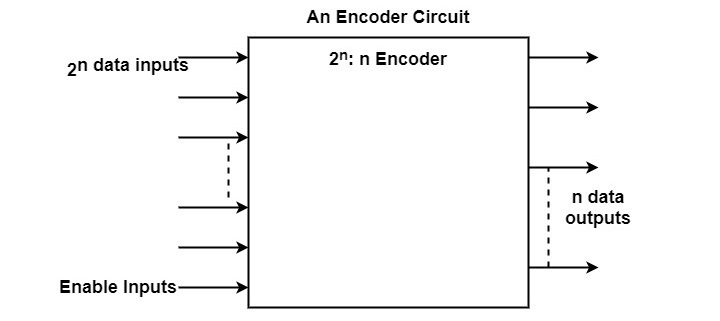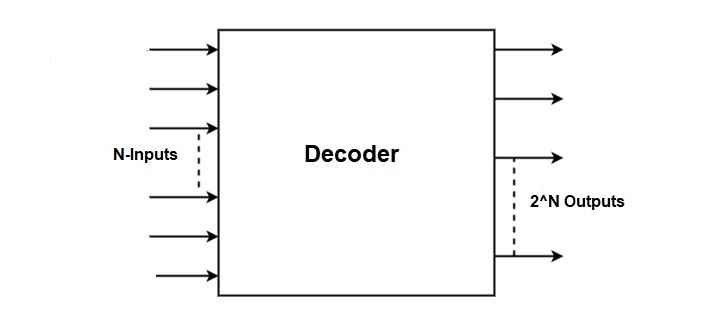# Difference between Encoder and Decoder

A combinational circuit is one that contains logic gates. Encoders and decoders are such combinational circuits in which one changes the input data into binary code and the other one decodes it to its original input signal. Both encoders and decoders are multiple input and multiple output devices

Encoding allows the input signal to function on various systems. For instance, when we search something in the web browser, it is converted into a binary code (encoding) which can be understood by it then search for it and send the results back to the user by converting the binary form into its original form (decoding).

## What is an ENCODER?

Encoder is a device which converts an original message into a coded message. It is a combinational circuit that applies logic on the input signal and convert it into a binary code.

Encoder takes the input signals and perform operations such as addition, subtraction, multiplication or division converting it into a Binary coded Decimal (BCD).

The input is given in multiple lines generally represented as 2n input lines. Here, 2n represents the maximum number of input lines it can encode. Then some logic is applied on them and convert it into a binary code of n output lines. These output lines act as a source of input lines for decoders.

Encoders are present at the message transmitting ends. It converts analog signals into digital signals. The OR logical gate is used in encoders to apply logic.

The main purpose of encoding data is to convert it in such a format so that the other systems finds it easy to use. The three main kinds of encoding are visual encoding, acoustic encoding, and semantic encoding.

Encoders converts normal codes to binary codes like −

• Decimal to binary (8x3)

• Octal to binary (10x4)

Encoders are used in video encoders, E-mail etc.,

Diagrammatic representation of Encoders is given below −• Data size is reduced

• Less memory required

• Data can’t be accessed by others unless they know the algorithm used hence providing security.

• Input time of data is less so large amounts of data can be added fastly within a short period of time

• Data entry accuracy is increased

• Data is fetched faster

• Data redundancies can be removed

## What is a Decoder?

Decoder is a device that converts the binary code signals into its original form. It is a combinational circuit that reverses the encoder operation.

The output of Encoder, that is binary coded Decimal (BCD) is the input for the decoder. It removes the logic encoded by the encoder and converts it into the original signal.

The input is given in n number of lines. This then decodes it and gives the output in 2n lines. Here, 2n represents the AND logical gate is used by decoders. Some of the decoders also use NAND gate. Decoders are complex to understand as they have to understand the logic applied by the encoder.

Decoders are installed at receiving ends. Decoders coverts the digital signals into analog signals.

Decoders converts binary code to other codes like −

• Binary to decimal (3x8)

• Binary to octal (4x10)

Decoders are used in microprocessors, memory chips etc., They are also mostly used in memory systems of computers.

Diagrammatic representation of Decoder is given below −## Encoder vs Decoder

The following table highlights the major differences between Encoders and Decoders −

Parameter

Encoder

Decoder

Definition

A combinational circuit that converts input signals into binary code

A combinational circuit which converts the binary code into original signals

No. of input lines

2n number of input lines are given

n number of input lines are taken

No. of output lines

n number of output lines

2n number of output lines

Logical gate

OR logical gate is used

A combination of AND gate and NOT gate are used

Input signal

It takes the actual message as input

It takes encoded binary code as input

Output signal

Output is given in the form of a binary code

Decoder gives the original message signal as output

Operation

It is very simple process

It is a complex process

Installation

Encoders are installed at the message transmitting end

Decoders are present at the receiving end

Signal conversion

Encoders convert analog signals into digital signals

Decoders convert digital signals into analog signals

Function

Encoders converts normal codes to binary codes like −

• Decimal to binary (8x3)

• Octal to binary (10x4)

Decoders converts binary code to other codes like −

• Binary to decimal (3x8)

• Binary to octal (4x10)

Applications

Encoders are used in video encoders, emails, etc.,

Decoders are used in microprocessors, memory chips, etc.,

## Conclusion

Both encoder and decoder are multiple input and multiple output devices and combinational logic circuits. Encoder encodes the actual data into binary code whereas decoder decodes the encoded data, i.e., binary code to get the original data signal.

Encoding is a writing process and decoding is a reading process. Encoders and decoders are opposite to each other in their functionality.

Updated on: 18-Apr-2023

3K+ Views